# Coefficient of friction facts for kids

Kids Encyclopedia Facts

A coefficient of friction is a value that shows the relationship between two objects and the normal reaction between the objects that are involved. It is a value that is sometimes used in physics to find an object's normal force or frictional force when other methods are unavailable.

The coefficient of friction is shown by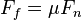$F_f = \mu F_{n}\,$ . In that equation,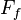$F_f$ is the frictional force,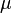$\mu$ is the coefficient of friction, and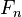$F_{n}\,$ is the normal force.

The coefficient$\mu$ can be two different things. It is either the coefficient of static friction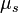$\mu_s$, or the coefficient of Dynamic friction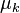$\mu_k$.The coefficient of static friction is the friction force between two objects when neither of the objects is moving. The coefficient of dynamic friction is the force between two objects when one object is moving, or if two objects are moving against one another.

The coefficient of friction is dimensionless and it does not have any unit. It is a scalar, meaning the direction of the force does not affect the physical quantity.

The coefficient of friction depends on the objects that are causing friction. The value is usually between 0 and 1 but can be greater than 1. A value of 0 means there is no friction at all between the objects; such is possible with Superfluidity. All objects, otherwise, will have some friction when they touch each other. A value of 1 means the frictional force is equal to the normal force. It is a misconception that the coefficient of friction is limited to values between zero and one. A coefficient of friction that is more than one just means that the frictional force is stronger than the normal force. An object such as silicone rubber, for example, can have a coefficient of friction much greater than one.

The friction force is the force exerted by a surface when an object moves across it - or makes an effort to move across it.

The frictional force or force of friction (static or kinetic) can be expressed as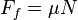$F_f=\mu N$ (1)

where$F_f$ is the frictional force (in Newtons),$\mu$ is the static ($\mu_s$) or kinetic ($\mu_k$) frictional coefficient (dimensionless) and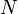$N$ is the normal force (in Newtons).

it could also be known as a friction.It is represented as(f).